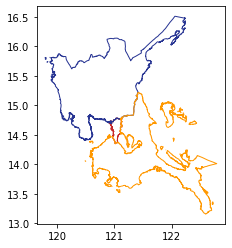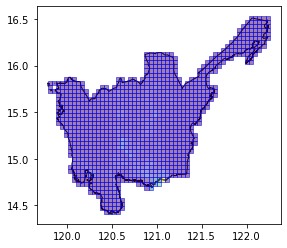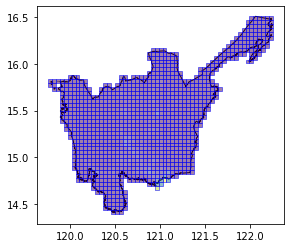A basic introduction to Raster Zonal Stats

## Basic Usage

Generate zonal stats for a GeoDataframe containing areas of interest using raster data

Terms:

• aoi - (area of interest) a geodataframe which we are interested in generating zonal statistics for
• raster data - the source raster containing the features which we are interested in collecting zonal stats for our aoi.
import geopandas as gpd
import matplotlib.pyplot as plt
import geowrangler.raster_zonal_stats as rzs


Our areas of interest (AOI) are three (3) Level 1 administration regions (ADM1) of the Philippines located in the island of Luzon.

%%time
# area multipolygons for regions 3,4,ncr of the philippines

CPU times: user 3.26 s, sys: 195 ms, total: 3.45 s
Wall time: 3.44 s

ax = aoi.plot(
ax=plt.axes(),
facecolor="none",
edgecolor=[
"#C62828",
"#283593",
"#FF9800",
],
)aoi

Reg_Code Reg_Name Reg_Alt_Name geometry
0 130000000 National Capital Region NCR MULTIPOLYGON (((121.03842 14.78525, 121.03815 ...
1 030000000 Region III Central Luzon MULTIPOLYGON (((120.11687 14.76309, 120.11684 ...
2 040000000 Region IV-A Calabarzon MULTIPOLYGON (((122.72165 13.36485, 122.72143 ...

We download our raster Data as GeoTiff files from the Humanitarian Data Exchange site.

phil_pop_link = "https://data.worldpop.org/GIS/Population/Global_2000_2020/2020/PHL/phl_ppp_2020.tif"
phil_pop_dset = "phl_pop_2020.tif"

%%time

![ ! -e ../data/{phil_pop_dset} ] && curl -o ../data/{phil_pop_dset} {phil_pop_link}

  % Total    % Received % Xferd  Average Speed   Time    Time     Time  Current
100  180M  100  180M    0     0   576k      0  0:05:19  0:05:19 --:--:--  430k
CPU times: user 12 s, sys: 3.57 s, total: 15.6 s
Wall time: 5min 19s


To create our raster zonal stats, we just need to set the aggregations, as well as some extra arguments, such as the nodata value in the raster.

%%time
results = rzs.create_raster_zonal_stats(
aoi,
f"../data/{phil_pop_dset}",
aggregation=dict(
func=["sum", "count"],
column="population",
output=["population_count", "samples"],
),
extra_args=dict(nodata=-99999),  # nodata value is -99999
)

CPU times: user 4.22 s, sys: 128 ms, total: 4.35 s
Wall time: 4.34 s

results

Reg_Code Reg_Name Reg_Alt_Name geometry samples population_count
0 130000000 National Capital Region NCR MULTIPOLYGON (((121.03842 14.78525, 121.03815 ... 70786 13165866.0
1 030000000 Region III Central Luzon MULTIPOLYGON (((120.11687 14.76309, 120.11684 ... 2558377 11493727.0
2 040000000 Region IV-A Calabarzon MULTIPOLYGON (((122.72165 13.36485, 122.72143 ... 1876244 15952383.0

### Using Grid Tile AOIs

We can also use tile grids as our AOIs.

grid_aoi_file = "../data/region3_admin_grids.geojson"

%%time
grid_aoi_results = rzs.create_raster_zonal_stats(
grid_aoi_file,
f"../data/{phil_pop_dset}",
aggregation=dict(
func=["sum", "count"],
column="population",
output=["population_count", "samples"],
fillna=[True, True],
),
extra_args=dict(nodata=-99999),  # nodata value is -99999
)

CPU times: user 8.57 s, sys: 12.2 ms, total: 8.59 s
Wall time: 8.58 s

grid_aoi_results.head()

x y geometry samples population_count
0 0 30 POLYGON ((119.78583 15.70870, 119.83075 15.708... 171 1171.764038
1 0 31 POLYGON ((119.78583 15.75193, 119.83075 15.751... 329 278.567200
2 0 32 POLYGON ((119.78583 15.79516, 119.83075 15.795... 345 279.140198
3 1 30 POLYGON ((119.83075 15.70870, 119.87566 15.708... 158 808.681152
4 1 32 POLYGON ((119.83075 15.79516, 119.87566 15.795... 20 0.000000
ax = aoi[aoi.Reg_Name == "Region III"].plot(
ax=plt.axes(), facecolor="none", edgecolor="black"
)
ax = grid_aoi_results.plot(
ax=ax, column="population_count", edgecolor="blue", alpha=0.5
)### Using Bing Tile Grid Tile AOIs

We can also use pre-existing Bing tile grids as our AOIs.

bingtile_grid_aoi_file = "../data/region3_bingtile_grid13.geojson"

%%time
bingtile_grid_aoi_results = rzs.create_raster_zonal_stats(
bingtile_grid_aoi_file,
f"../data/{phil_pop_dset}",
aggregation=dict(
func=["sum", "count"],
column="population",
output=["population_count", "samples"],
fillna=[True, True],
),
extra_args=dict(nodata=-99999),  # nodata value is -99999
)

CPU times: user 10.6 s, sys: 124 ms, total: 10.7 s
Wall time: 10.7 s

bingtile_grid_aoi_results.head()

ax = aoi[aoi.Reg_Name == "Region III"].plot(